## Boolean Function

A Boolean function invariables is a function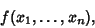where each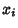can be 0 or 1 and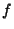is 0 or 1. Determining the number of monotone Boolean functions ofvariables is known as Dedekind's Problem. The number of monotonic increasing Boolean functions ofvariables is given by 2, 3, 6, 20, 168, 7581, 7828354, ... (Sloane's A000372, Beeler et al. 1972, Item 17). The number of inequivalent monotone Boolean functions ofvariables is given by 2, 3, 5, 10, 30, ...(Sloane's A003182).

Let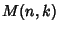denote the number of distinct monotone Boolean functions ofvariables with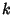mincuts. Then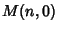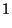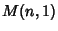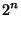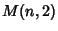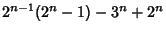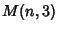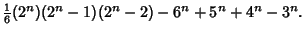References

Beeler, M.; Gosper, R. W.; and Schroeppel, R. HAKMEM. Cambridge, MA: MIT Artificial Intelligence Laboratory, Memo AIM-239, Feb. 1972.

Sloane, N. J. A. Sequences A003182/M0729 and A000372/M0817 in An On-Line Version of the Encyclopedia of Integer Sequences.'' http://www.research.att.com/~njas/sequences/eisonline.html and Sloane, N. J. A. and Plouffe, S. The Encyclopedia of Integer Sequences. San Diego: Academic Press, 1995.